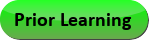Sep 21, 2023
2021-2022 College Catalog
 Select a Catalog 2023-2024 College Catalog 2022-2023 College Catalog [ARCHIVED CATALOG] 2021-2022 College Catalog [ARCHIVED CATALOG] 2020-2021 College Catalog [ARCHIVED CATALOG] 2019-2020 College Catalog [ARCHIVED CATALOG] *** DRAFT 2024-2025 College Catalog DRAFT *** [ARCHIVED CATALOG]
 HELP 2021-2022 College Catalog [ARCHIVED CATALOG] Print-Friendly Page (opens a new window) Add to Portfolio (opens a new window)

# MAT 189 - Precalculus II [SUN# MAT 1187]

3 Credits, 3 Contact Hours
3 lecture periods 0 lab periods

Continuation of MAT 188 . College-level trigonometry. Includes trigonometric functions, angle measure, graphs, identities, equations, polar coordinates, conic sections, and calculator use. May also include parametric equations, vectors, and complex numbers.

Prerequisite(s): Within the last three years: MAT 188  with a grade of C or better, or required score on the mathematics assessment test.
Recommendation: This course is intended as an intensive preparation for students who plan to continue with Calculus.
Information: No more than 7 credits may be applied toward graduation from the following list of courses: MAT 151 , MAT 188 , and MAT 189. A graphing calculator is required for this course and will be used extensively.
Gen-Ed: Meets AGEC - MATH; Meets CTE - M&S.Course Learning Outcomes
1. Solve trigonometric equations and verify trigonometric identities.
2. Graph trigonometric functions polar equations, and conic sections.
3. Solve triangles and real world applications.

Performance Objectives:
1. Convert between radians and degrees measures.
2. Define, graph, and evaluate the six trigonometric functions and their inverses.
3. Solve trigonometric equations algebraically and graphically.
4. Use trigonometric identities to simplify expressions and solve equations.
5. Graph polar equations; convert between rectangular and polar coordinates.
6. Use the standard equations for conic sections and sketch their graphs; identify types of conic sections and determine their features.
7. Use a graphing calculator to evaluate, graph, and analyze functions.
8. Solve application problems.

Outline:
1. Trigonometric Functions
1. Angle measure
2. Definition and graphs of the six trigonometric functions
3. Trigonometric Identities
1. Basic, Pythagorean and Reciprocal
2. Sum and difference formulas
3. Double angle and half angle formulas
4. Sum to product and product to sum (optional)
5. Verification of identities
4. Inverse trigonometric functions
5. Law of Sines and Law of Cosines
6. Trigonometric Equations
7. Applications
2. Polar Coordinates
1. Conversion between rectangular and polar coordinates
2. Graphs of polar equations
3. Conic Sections
1. Standard equations: Parabolas, Circles, Ellipses
2. Identification of type of conic section
3. Determination of features: center, vertices
4. Optional:
1. Hyperbolas
2. Determination of foci, asymptotes
4. Calculator Use
1. Numerical calculations and evaluation of functions
2. Graphs and analysis of functions
5.  Additional Topics: select at least one
1. Complex Numbers
1. Definition and graphical representation
2. Operations
3. Conversion between standard form and polar form
4. DeMoivre’s Theorem
2. Vectors